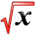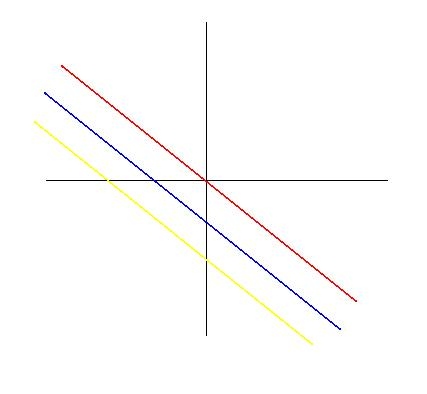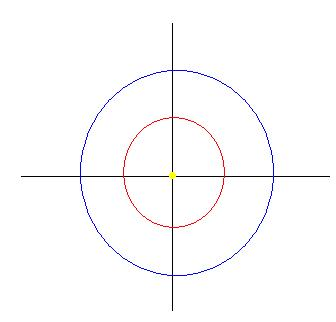# Level CurvesThis article is part of the MathHelp Tutoring Wiki

Example 1:
Question: Find the level curves of heights C = -1, 0, 1 for f(x,y) = x+y+1
Solution:

For height C = -1 ( Yellow Line )
f(x,y) = x+y+1 = -1
x+y+1 = -1
y = -2-x
slope = -1
y-intercept = -2
For height C = 0 ( Blue Line )
x+y+1 = 0
y = -1-x
For height C = 1 ( Red Line )
x+y+1 = 1
y = -x

This is the graph that refers to Example 1.Example 2:
Question: Find the level curves of heights C = 4, 1, 0, and -1 for f(x,y) = $x^{2}+y^{2}$Solution:

For height C = 4 ( Blue Circle )
$x^{2}+y^{2}=4$$x^{2}+y^{2}=2^{2}$For height C = 1 ( Red Circle )
$x^{2}+y^{2}=1$$x^{2}+y^{2}=1^{2}$For height C = 0 ( Yellow Dot )
$x^{2}+y^{2}=0$Only solution is x = 0, y = 0.
For height C = -1
$x^{2}+y^{2}=-1$Does not exist because $x^{2}+y^{2}$has to be greater than equal to 0.

This is the graph that refers to Example 2.